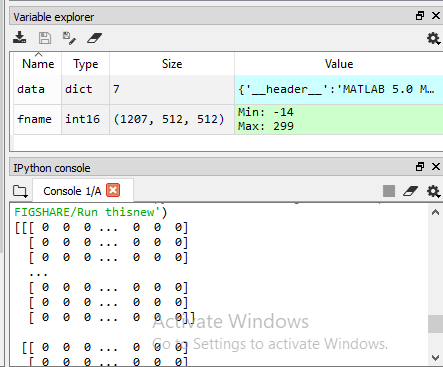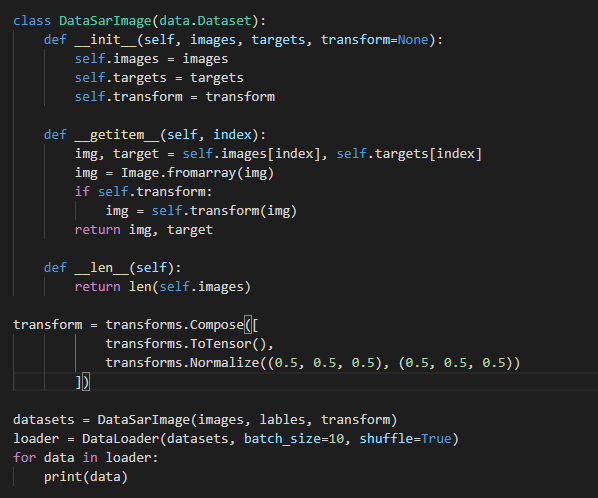# How to convert .mat file struct into pytorch Tensors

Hi ,I have 3064 images dataset into single .mat (matlab) file.
The file has structures format.
How can I access the structure using pytorch.I have Loaded in Python the .mat file but not sure how to access data from it.
Following are the items in cjdata structure
cjdata.image
cjdata.id
cjdata.tumorborder

1 Like

I guess you’ve opened the file using `scipy.io`?
If so, you should get a `dict` with all the members and can just load these arrays as numpy arrays.
Once you have the numpy data, you can transform them to `torch.Tensors` using `torch.from_numpy()`.
Have a look at some examples from the scipy docs on how to load the data.

1 Like

I need little help more please.
this is how is combined all .mat structure in one mat.See so you can get idea for my problem.

``````% % Making single mat file for all images detail
% for i=1:3064
%     fname = strcat('data/figshareBrainTumorDataPublic/',num2str(i),'.mat')
%     label(i) = cjdata.label;
%     PID(i) = {cjdata.PID};
%     if (size(cjdata.image,1) ~= 512)
%         image(i,:,:) = imresize(cjdata.image, [512, 512]);
%     else
%         image(i,:,:) = cjdata.image;
%     end
%     clear cjdata fname
% end
% save('data/figshareBrainTumorDataPublicNewSingle/data.mat', 'label', 'PID', 'image', 'tumorMask');
``````

The code in Python so far is :

``````import scipy.io as io
fname='./data.mat'
``````

‘image’ has all 3064 images.How can I access one image from it :fname=data[‘image’] or selective images.like 4 image.Thankyou for helping me
Please suggest best way to make the data ready for pytorch CNN.I m beginner at this.1 Like

Is `data['image']` returning a numpy array?
If so, you could just slice it using e.g. `data['image'][:4]`, if you want to get the first 4 images.
You could try to write your custom `Dataset`, load all data in `__init__`, and get a single sample in `__getitem__`. Here is some dummy example:

``````class MyDataset(Dataset):
def __init__(self, mat_path):
self.images = torch.from_numpy(data['images'])

def __getitem__(self, index):
x = self.images[index]
y = self.targets[index]
return x, y

def __len__(self):
return len(self.images)
``````

Also, have a look at the Data loading tutorial to see how the `Dataset` and `DataLoader` play together.

Is `imshow` showing something?
Based on the variable explorer it looks like your first images are all zero.
Also, the images seem to be single channel images. Is this correct?

Imshow is not showing anything.yes you are right.Your command data[‘image’][:4] return (4,512,512) and does not displays images.
But `fname=data['image']`
returns long arrays.Are you expecting this image format? In a previous post you’ve mentioned `data['image']` should contain 3064 images. Now your `fname` seems to have 1207 images. Maybe the data is being stored/loaded in the wrong format.

I don’t know why the it has 1207 images.I have stored through loop with i value 3064.
Why this happening I don’t know.This is how i stored in one mat file:

``````% % Making single mat file for all images detail
for i=1:3064
fname = strcat('data/figshareBrainTumorDataPublic/',num2str(i),'.mat')
label(i) = cjdata.label;
PID(i) = {cjdata.PID};
image(i,:,:) = cjdata.image;
tumorBorder(i,:) = cjdata.tumorBorder;
end
% save('data/figshareBrainTumorDataPublicNewSingle/data.mat', 'label', 'PID', 'image', 'tumorMask');
save('data/figshareBrainTumorDataPublicNewSingle/data.mat', 'label', 'PID', 'image', 'tumorBorder', 'tumorMask');

``````

By the way I have the other method running byside.saved 3064 images as png from mat files.I have saved 3 labeled folders (images stored as PNG) .But found RuntimeError: Found 0 files in subfolders.

.
"each subfolders in "train “have png images.”

Hi Ptrblck

I want to save data as mat file in the pytorch which command is the right one. I can load mat file but now I need to save my tensor or numpy array as mat file.
I used this one >>> scipy.io.savemat(‘images1.mat’, images1.numpy())
images1 are my input patches. but it dose not work.

The command looks alright.
What kind of error do you get?

Thank you I solve it I should have used

io.savemat(os.path.join(root_dirDurringTraining11+’/’,“images”+str(i)+".mat"), {“images11”: images1.numpy()})

Hi, I got sing channel images from ‘.mat’. And I write my custom Dataset, but got some errors.RuntimeError: output with shape [1, 21, 21] doesn’t match the broadcast shape [3, 21, 21]my images array shape(1596, 21, 21)

@ptrblck sorry if I disturb you

I guess `transforms.Normalize` raises this error, since you are using a single-channel tensor, while `Normalize` uses 3 values for the `mean` and `stddev`.
If you are dealing with grayscale images, you could pass `tuples` containing a single element to `Normalize`:

``````transforms.Normalize((0.5,), (0.5,))
``````

thank you sir！ sovled it